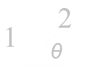﻿ Congruent Rectangles Puzzle v2# Congruent Rectangles Puzzle v2Last week, I wrote about a fun little geometry puzzle. Here it is again: Imagine you have a unit square. From one edge you mark off a rectangle. If it is possible to place an identical (congruent) rectangle at an angle inside the complementary part of the square, what is the width of these rectangles? The solution I published involved equating the areas of the different shapes. A few of you* wrote in suggesting alternative (simpler?) solutions involving some basic trigonometry. This posting is a hybrid of these. *Special shout outs to: Stephen Li, Javier Romualdez, Scott Carr and George Xie.

You can see details of my solution last week here.

### Trigonometric Solution

As before, we'll define the width of the rectangle to be x and we'll also define the angle the second rectangle makes with the horizontal to be θ. We can mark similar angles on the diagram.

 We can create two equations for x in terms of θ by summing up the edges of the square (vertically and horizontally) and equating these to one (the width of the square).Equating these two equations and simplifying:Using what was (probably) the second trig identity you ever learned (the first being sinθ/cosθ=tanθ)We can can simplify and reduce down to this:This important result tells us the shape of the triangle. Using Pythagoras we can determine the adjacent edge ratio and thus cosθ

### Result

Inserting the values for cosθ and sinθ back into equation 1, we get the answer:Thankfully, this is the same answer as the one we obtained by the (slightly more convoluted) method of conservation of area

You can find a complete list of all the articles here.Click here to receive email alerts on new articles.